# CREST Mathematics Olympiad Class 6 Sample Papers

REGISTER NOW

## Syllabus:

Section 1: Knowing Our Numbers, Whole Numbers, Playing with Numbers, Basic Geometrical Ideas, Understanding Elementary Shapes, Integers, Fractions, Decimals, Data Handling, Mensuration, Algebra, Ratio And Proportion, Symmetry, Practical Geometry.

Achievers Section: Higher Order Thinking Questions - Syllabus as per Section 1

 Q.1 George travels from place A to place B and Peter travels from place B to place A. George travels one-third of the distance and Peter travels one-fourth of the distance. The distance travelled by George is one kilometre more than the distance travelled by Peter. What is the distance between place A and place B? (In km)
 Q.2 In the given rectangle MNOP, if length is decreased by 3x, then find the new perimeter: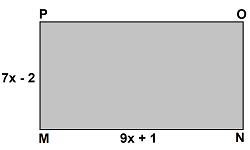Q.3 The bar graph shows the sale of chocolates in 5 months from January to May: What is the total sale of chocolates from January to May?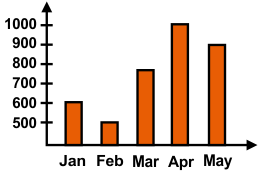Q.4 What will come in place of "?":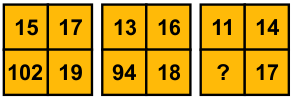Q.5 In the given figure ∠1 = x and ∠7 = 2x/3. Find the value of ∠5: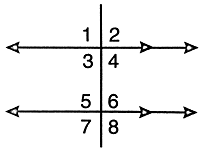Q.6 How many lines of symmetry are there in an equilateral triangle?
 Q.7 Two regular pentagons of side 5 cm are joined together as shown in the figure. Find the perimeter of the new figure: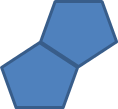Q.8 Fill in the blank: A quadrilateral with only one pair of opposite side parallel is called a __________
 Q.9 Which of the following will not be the statement for the algebraic expression 20z?
 Q.10 In the figure shown below, ABC and EFC are straight lines. Find the value of x + y: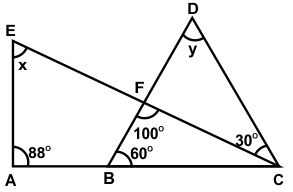Sample PDF of CREST Mathematics Olympiad for Class 6: